# RS Aggarwal Class 8 Solutions Chapter 14 Polygons Ex 14A

## RS Aggarwal Class 8 Solutions Chapter 14 Polygons Ex 14A

These Solutions are part of RS Aggarwal Solutions Class 8. Here we have given RS Aggarwal Solutions Class 8 Chapter 14 Polygons Ex 14A.

Other Exercises

Question 1.
Solution:
We know that sum of exterior angles of a polygon is 360°
Then,
(i) Pentagon’s exterior angle = $$\frac { { 360 }^{ o } }{ 5 }$$
= 72°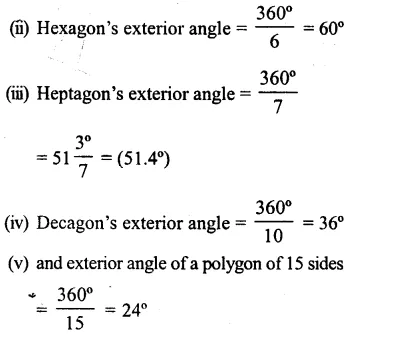Question 2.
Solution:
Each exterior angle a n sided polygon = 50°
No of sides = $$\frac { { 360 }^{ o } }{ 50 }$$
= $$7\frac { 1 }{ 5 }$$
Which is not possible to have $$7\frac { 1 }{ 5 }$$ sides
Which is not a whole number

Question 3.
Solution:
We know that each interior angle of a regular polygon of n sides = $$\\ \frac { 2n-4 }{ n }$$ right angle
(i) Polygon having 10 sides, each interior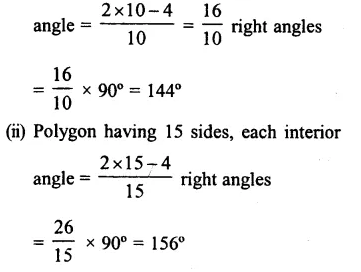Question 4.
Solution:
Let interior angle of a polygon having n sides = 100°
$$\\ \frac { 2n-4 }{ n }$$ x 90° = 100°
=>$$\\ \frac { 2n-4 }{ n }$$
= $$\\ \frac { 100 }{ 90 }$$
= $$\\ \frac { 10 }{ 9 }$$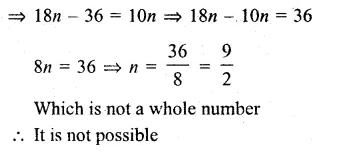Question 5.
Solution:
We know that sum of all interior angles = 2n – 4 right angles
(i) Pentagon
Sum of its angles = (2 x 5 – 4) x 90°
= 6 x 90° = 540°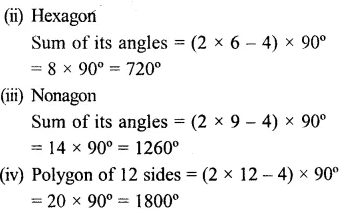Question 6.
Solution:
We know that number of diagonal of polygon having n sides = $$\frac { n\left( n-3 \right) }{ 2 }$$
(i) In heptagon, no of diagonals = $$\frac { 7\left( 7-3 \right) }{ 2 }$$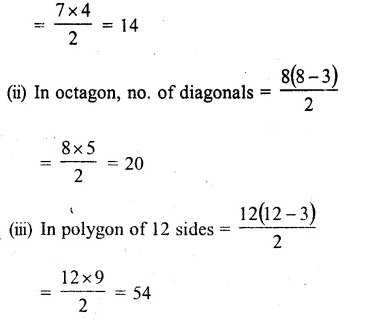Question 7.
Solution:
We know that each exterior angle
= $$\frac { { 360 }^{ o } }{ n }$$
Where n sides are of polygon
(i) Each exterior angle = 40°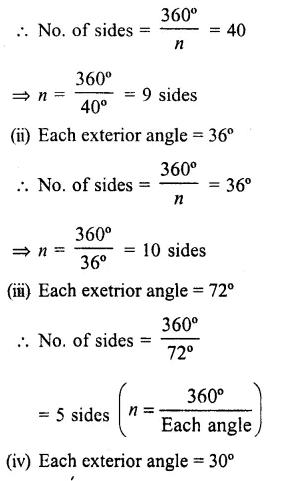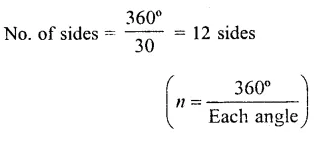Question 8.
Solution:
We know that sum of all exterior angle of a polygon – 360°
Exterior ∠A + ∠B + ∠C + ∠D = 360°
=> 115° + x + 90° + 50° = 360°
=> 255° + x + 360°
=> x = 360° – 255°
=> x = 105°

Question 9.
Solution:
In the given figure polygon is of 5 sides and each interior angle is x
$$x=\frac { 2x-4 }{ n } \times { 90 }^{ o }=\frac { 2\times 5-4 }{ 5 } \times { 90 }^{ o }$$
= $$=\frac { 6 }{ 5 } \times { 90 }^{ o }$$
= 108°

Hope given RS Aggarwal Solutions Class 8 Chapter 14 Polygons Ex 14A are helpful to complete your math homework.

If you have any doubts, please comment below. Learn Insta try to provide online math tutoring for you.